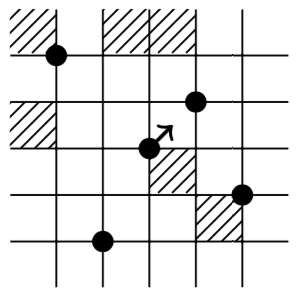# Wilf-classification of mesh patterns of short length

### Electronic Journal of Combinatorics, Volume 22 (2015)The goal of this paper is to Wilf-classify mesh patterns of length 2. To this end we prove The Shading Lemma, which gives sufficient conditions for two mesh patterns to be coincident (i.e., avoided by the same permutations) and therefore Wilf-equivalent. The lemma, along with other rules which implie Wilf-coincidence we show that there are at most 56 Wilf-classes of mesh patterns of length 2. We conjecture that the exact number is 46 and hope to revisit this area and prove this conjecture.

Some of the conjectures in the paper have been proven in follow-up papers. Specifically, the paper Distributions of mesh patterns of short lengths, by Kitaev and Zhang, resolves some of the conjectures in Tables 11 and 12: They prove that the sets of patterns {48, 49}, {55 and 56}, {63, 64, 65} are equidistributed, and therefore Wilf-equivalent.

See also Distributions of several infinite families of mesh patterns, by Kitaev, Zhang and Zhang, which proves several distribution results of mesh patterns.